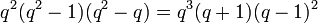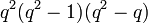Element structure of general affine group of degree two over a finite field

(diff) ← Older revision | Latest revision (diff) | Newer revision → (diff)
This article gives specific information, namely, element structure, about a family of groups, namely: general affine group of degree two.
View element structure of group families | View other specific information about general affine group of degree two

This article gives the element structure of the general affine group of degree two over a finite field. Similar structure works over an infinite field or a field of infinite characteristic, with suitable modification. For more on that, see element structure of general affine group of degree two over a field.

The discussion here builds upon the discussion of element structure of general linear group of degree two over a finite field.

Summary

Item Value
order$q^2(q^2 - 1)(q^2 - q) = q^3(q + 1)(q - 1)^2$
exponent  ?
number of conjugacy classes$q^2 + q - 1$

Particular cases$q$ (field size)$p$ (underlying prime, field characteristic) general affine group$GL(2,q)$ order of the group (=$q^2(q^2 - 1)(q^2 - q)$) number of conjugacy classes (=$q^2 + q - 1$) element structure page
2 2 symmetric group:S4 24 5 element structure of symmetric group:S4
3 3 general affine group:GA(2,3) 432 11 element structure of general affine group:GA(2,3)
4 2 general affine group:GA(2,4) 2880 19
5 5 general affine group:GA(2,5) 12000 29